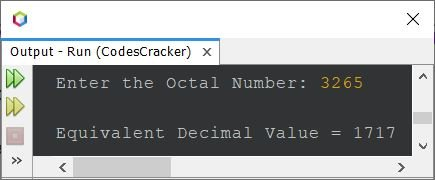# Java Program to Convert Octal to Decimal

This article covers a program in Java that converts a given octal number, by user at run-time of the program, to its equivalent decimal value.

If you're not aware about, how the octal to decimal conversion takes place, then refer to Octal to Decimal. Now let's create the program.

## Octal to Decimal in Java

The question is, write a Java program to convert octal number to decimal. The octal value must be received by user at run-time. The program given below is its answer:

```import java.util.Scanner;
import java.lang.Math;

public class CodesCracker
{
public static void main(String[] args)
{
int octal, decimal=0, i=0, rem;
Scanner s = new Scanner(System.in);

System.out.print("Enter the Octal Number: ");
octal = s.nextInt();

while(octal!=0)
{
rem = octal%10;
decimal = (int) (decimal + (rem*Math.pow(8, i)));
i++;
octal = octal/10;
}

System.out.println("\nEquivalent Decimal Value = " +decimal);
}
}```

The sample run of above program with user input 3207 as octal number to convert and print its equivalent decimal value, is shown in the snapshot given below:Here is another version of the same program. This program uses for loop, instead of while. Also, this program does not uses Math.pow() method.

```import java.util.Scanner;

public class CodesCracker
{
public static void main(String[] args)
{
int octal, decimal, i;
Scanner s = new Scanner(System.in);

System.out.print("Enter the Octal Number: ");
octal = s.nextInt();

for(i=0, decimal=0; octal!=0; octal /= 10, i++)
{
int m=1;
for(int k=1; k<=i; k++)
m *= 8;
decimal = (int) (decimal+((octal%10)*m));
}

System.out.println("\nEquivalent Decimal Value = " +decimal);
}
}```

Here is its sample run with user input 3265#### Same Program in Other Languages

Java Online Test

« Previous Program Next Program »